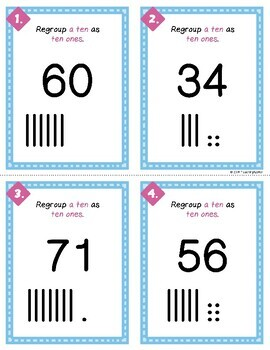# Regrouping for Subtraction: Regroup a Ten as Ten Ones1st - 4th, Homeschool
Subjects
Standards
Resource Type
Formats Included
• Zip
Pages
29 pages

#### Also included in

1. This 72 page BUNDLE will make sure your students have a solid understanding of regrouping for subtraction. Students will regroup tens, hundreds, and across zeroes. If students don't understand what happens mathematically when they regroup, subtraction with regrouping can end up overwhelming!Let's s
\$7.00
\$8.00
Save \$1.00
2. This 144 page MEGA BUNDLE will make sure your students understanding regrouping to prepare for both addition and subtraction. It includes work mats, task cards, worksheets, journals and PPTs. Included in this Product:Regrouping for Subtraction104 Task Cards = 52 Task Cards (x2 Different Versions)Reg
\$12.00
\$15.50
Save \$3.50

### Description

If students don't understand why regrouping a ten as ten ones makes mathematical sense, subtraction with regrouping can be overwhelming! This product will make sure your students have a solid understanding that one ten is the same as ten ones.

Included in this Product:

• Student Reference Cards: 1 Ten = 10 Ones
• Student Work Mat
• 20 Task Cards **2 Versions**
• With Place Value Pictures (Answers Included)
• Scoot Recording Page (with Answer Key)
• How to Regroup Sequencing Cut and Paste Activity
• PPT with Teacher Script - Building Mental Models
• 1 Worksheet
• 5 Student Journal Prompts

Let's stay connected! Click to ((( FOLLOW MY STORE )))

------------------------------------------------------------------------------------------

Interested in other math topics?

MEGA BUNDLE - Regrouping for Addition and Subtraction

BUNDLE - Regrouping for Subtraction

Centimeter and Inch Measurement - Building Number Sense

Comparing 3 Digit Numbers using Place Value

Total Pages
29 pages
N/A
Teaching Duration
N/A
Report this Resource to TpT
Reported resources will be reviewed by our team. Report this resource to let us know if this resource violates TpT’s content guidelines.

### Standards

to see state-specific standards (only available in the US).
100 can be thought of as a bundle of ten tens - called a “hundred.”
Fluently add and subtract within 100 using strategies based on place value, properties of operations, and/or the relationship between addition and subtraction.
Add and subtract within 1000, using concrete models or drawings and strategies based on place value, properties of operations, and/or the relationship between addition and subtraction; relate the strategy to a written method. Understand that in adding or subtracting three-digit numbers, one adds or subtracts hundreds and hundreds, tens and tens, ones and ones; and sometimes it is necessary to compose or decompose tens or hundreds.
Explain why addition and subtraction strategies work, using place value and the properties of operations.